# Samacheer Kalvi 9th Maths Solutions Chapter 9 Probability Ex 9.1

## Tamilnadu Samacheer Kalvi 9th Maths Solutions Chapter 9 Probability Ex 9.1

Question 1.
You are walking along a street. If you just choose a stranger crossing you, what is the probability that his next birthday will fall on a Sunday?
Solution:
Days in a week (S) = {Sunday, Monday, Tuesday, Wednesday, Thursday, Friday, Saturday}
n( S) = 7
∴ No. of days in week = 7
Event of selecting Sunday (A) = {Sunday}
n(A) = 1
∴ Probability of selecting Sunday = $$\frac{n(\mathrm{A})}{n(\mathrm{S})}=\frac{1}{7}$$Question 2.
What is the probability of drawing a King or a Queen or a Jack from a deck of cards?
Solution:
Number of cards n(S) = 52
No. of King cards n(A) = 4
No. of Queen cards n(B) = 4
No. of Jack cards n(C) = 4
Probability of drawing a King card
$$=\quad \frac{n(\mathrm{A})}{n(\mathrm{S})}=\frac{4}{52}$$
Probability of drawing a Queen card
$$=\frac{n(\mathrm{B})}{n(\mathrm{S})}=\frac{4}{52}$$
Probability of drawing a Jack card
$$=\frac{n(\mathrm{C})}{n(\mathrm{S})}=\frac{4}{52}$$
The Probability of drawing a King or a Queen or a Jack from a deck of cards
$$=\mathrm{P}(\mathrm{A})+\mathrm{P}(\mathrm{B})+\mathrm{P}(\mathrm{C})=\frac{4}{52}+\frac{4}{52}+\frac{4}{52}=\frac{4+4+4}{52}=\frac{12}{52}=\frac{3}{13}$$

Question 3.
What is the probability of throwing an even number with a single standard dice of six faces?
Solution:
Faces of a dice (S) = {1, 2, 3, 4, 5, 6}
n(S) = 6
Event of throwing an even number
A = {2, 4, 6}, n(A) = 3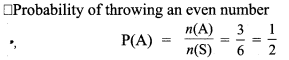Question 4.
There are 24 balls in a pot. If 3 of them are Red, 5 of them are Blue and the remaining are Green then, what is the probability of picking out
(i) a Blue ball,
(ii) a Red ball and
(iii) a Green ball?
Solution:
n(S) = 24
Red – n(R) = 3
Blue – n(B) = 5
Green – n(G) = 16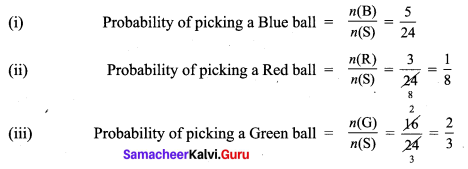Question 5.
When two coins are tossed, what is the probability that two heads are obtained?
Solution:
Sample space when two coins are tossed (S) = {HH, TT, HT, TH}
n(S) = 4
Event of getting two heads (A) = {HH}
n(A) = 1
Probability of getting two heads P(A) = $$\frac{n(\mathrm{A})}{n(\mathrm{S})}=\frac{1}{4}$$

Question 6.
Two dice are rolled, find the probability that the sum is
(i) equal to 1
(ii) equal to 4
(iii) less than 13
Solution:
When two dice are rolled Sample space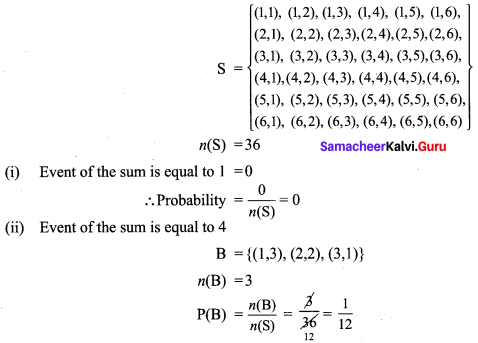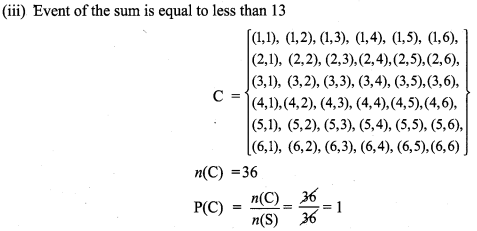Question 7.
A manufacturer tested 7000 LED lights at random and found that 25 of them were defective. If a LED light is selected at random, what is the probability that the selected LED light is a defective one.
Solution:
n(S) = 7000 S – Total no. of lights.
n(A) = 25 A – Defective ones.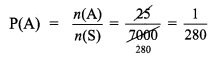Question 8.
In a football match, a goalkeeper of a team can stop the goal, 32 times out of 40 attempts tried by a team. Find the probability that the opponent team can convert the attempt into a goal.
Solution:
Total no. of attempts n(S) = 40
Total no. of attempts by A team n(A) = 32
Total no. of attempts by the opponent team B = n(B) = 40 – 32 = 8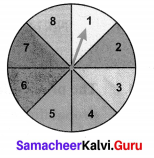Question 9.
What is the probability that the spinner will not land on a multiple of 3?
Solution:
Total no. of choices n(S) = 8
Total no. of multiples of 3 A = {3, 6}
n(A) = 2
Event of non-multiples of 3B = {1, 2, 4, 5, 7, 8}
n(B) = 6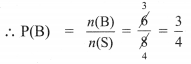Question 10.
Frame two problems in calculating probability, based on the spinner shown here.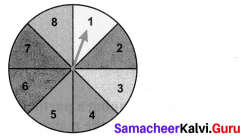Solution:
(i) What is the probability that the spinner will land on an even number?
(ii) What is the probability that the spinner will not land on a prime number.